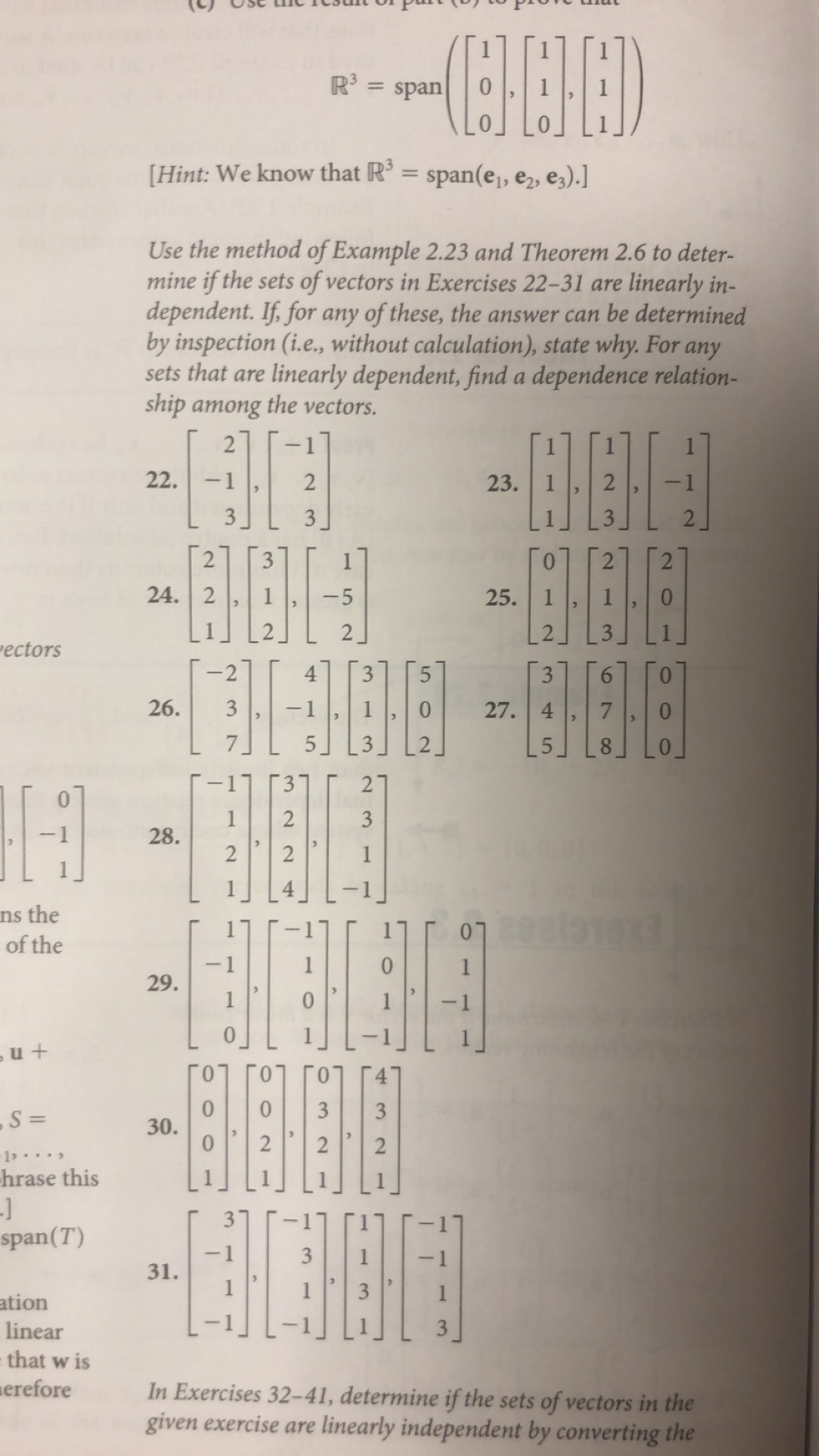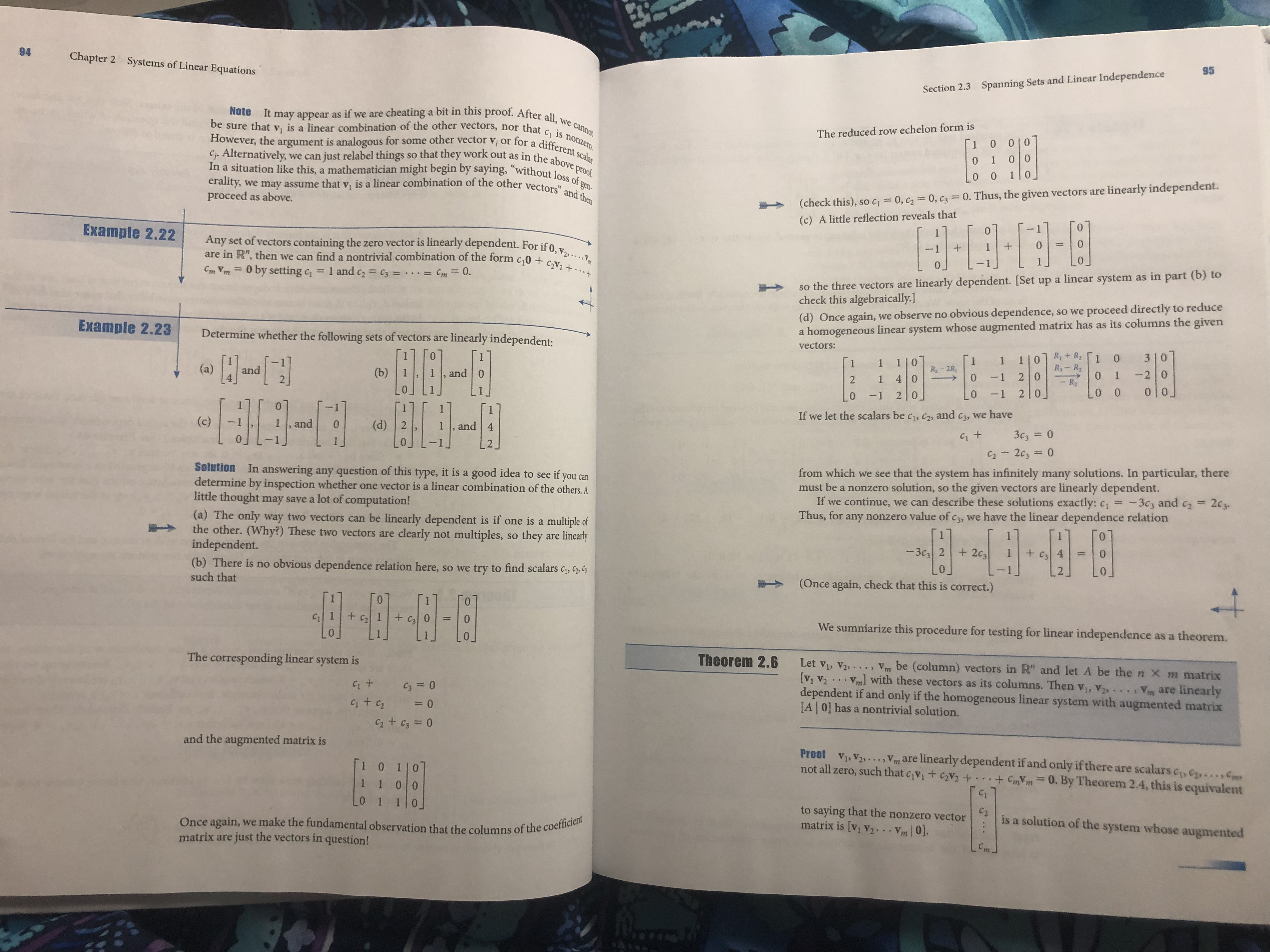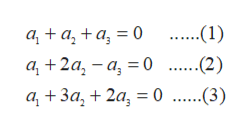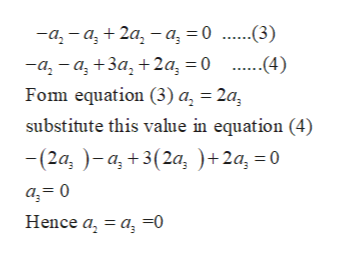R3spanHint: We know that R = span(e1, e2, e3).]Use the method of Example 2.23 and Theorem 2.6 to deter-mine if the sets of vectors in Exercises 22-31 aredependent. If, for any of these, the answer can be determinedby inspection (i.e., without calculation), state why. For anysets that arelinearly in-linearly dependent, find a dependence relation-ship among the vectors.2-122.2- 123.332232224. 225. 1-52"ectors2435326.27. 4-1532112328.22ns theof theFENELETA1913129ь и +1030.1033-S =21.hrase this-Jspan(T)3-1331.1ationlinear3that w isereforeIn Exercises 32-41, determine if the sets of vectors in thegiven exercise arelinearly independent by converting theLO21 94Chapter 2Systems of Linear Equations95Section 2.3Spanning Sets and Linear Independencecheating a bit in this proof. After all, we cannotbe sure that v, is a linear combination of the other vectors, nor that ci is nonzero.However, the argument is analogous for some other vector v or for a different scalar, we can just relabel things so that they work out as in the above proof.In a situation like this, a mathematician might begin by saying, "without loss of gen-we may assume that v, is a linear combination of the other vectors and thenNoteIt may appear as if we areThe reduced row echelon form is10 010Cf. Alternatively,0 10 000erality.proceed as above.linearly independent.(check this), soC0, c2= 0 , c3= 0. Thus, the given vectors are(c) A little reflection reveals that0Example 2.22Any set of vectors containing the zero vector is linearly dependent. For if 0, V2.are in R", then we can find a nontrivial combination of the form c 0 + Cv2+1+0so the three vectors are linearly dependent. [Set up a linear system as in part (b) tocheck this algebraically.]Cm Vm0 by setting c= 1 and c2c3 =.=Cm= 0.we observe no obvious dependenceso we proceed directly to reduce(d) Once again,homogeneous linear system whose augmented matrix has as its columns the givenExample 2.23aDetermine whether the following sets of vectors arelinearly independent:vectors:Ry+ R203|01R3- R011 | 01R-2R0111 |0(a)and22 01-2 0(b)and 0214 0-1-1 2|00 00 0-12 00If we let the scalars be ci, c2, and c3, we have(c)1,and0(d) 2and 43c3= 0-1J1J2C22c3= 0Solution In answering any question of this type, it is a good idea to see if you candetermine by inspection whether one vector is a linear combination of the others. Alittle thought may save a lot of computation!from which we see that the system has infinitely many solutions. In particular, theremust be a nonzero solution, so the given vectors areIf we continue, we can describe these solutions exactly: cThus, for any nonzero value of c3, we have the linear dependence relationlinearly dependent.-3c3 and c22c3.(a) The only way two vectors can be linearly dependent is if one is a multiple ofthe other. (Why?) These two vectors are clearly not multiples, so theyindependent.(b) There is no obvious dependence relation here, so we try to find scalars c, Csuch that0arelinearly-3c3 2+203+c3 402Y(Once again, check that this is correct.)00C1+ c1+ c3We summiarize this procedure for testing for linear independenceas a theorem.The corresponding linear system isTheorem 2.6Let vi, V2..., vm be (column) vectors in R" and let A be the n X m matrix[v1 V2dependent if and only if the homogeneous linear system with augmented matrix[A 0] has a nontrivial solution.with these vectors as its columns. Then v, VC3=0are linearly= 0C2 + C3= 0and the augmented matrix is1 0 1|01 1 0 0Proof Vi, V2.,Vm are linearly dependent if and only if there are scalars c, C,not all zero, such that cv + c2v2 + + CmVm= 0. By Theorem 2.4, this is equivalentC)LO 1 1|0.to saying that the nonzero vectormatrix is [v1 v2 . . Vm 0.Czis a solution of the system whose augmented, we make the fundamental observation that the columns of the coefficientjust the vectors in question!Once again,matrix arem

Question

#23help_outlineImage TranscriptioncloseR3 span Hint: We know that R = span(e1, e2, e3).] Use the method of Example 2.23 and Theorem 2.6 to deter- mine if the sets of vectors in Exercises 22-31 are dependent. If, for any of these, the answer can be determined by inspection (i.e., without calculation), state why. For any sets that are linearly in- linearly dependent, find a dependence relation- ship among the vectors. 2 -1 22. 2 - 1 23. 3 3 2 2 3 2 2 24. 2 25. 1 -5 2 "ectors 2 4 3 5 3 26. 27. 4 -1 5 3 21 1 2 3 28. 2 2 ns the of the FENELETA 1913 1 29 ь и + 10 30. 10 3 3 -S = 2 1. hrase this -J span(T) 3 -1 3 31. 1 ation linear 3 that w is erefore In Exercises 32-41, determine if the sets of vectors in the given exercise are linearly independent by converting the LO 2 1 fullscreenhelp_outlineImage Transcriptionclose94 Chapter 2 Systems of Linear Equations 95 Section 2.3 Spanning Sets and Linear Independence cheating a bit in this proof. After all, we cannot be sure that v, is a linear combination of the other vectors, nor that ci is nonzero. However, the argument is analogous for some other vector v or for a different scalar , we can just relabel things so that they work out as in the above proof. In a situation like this, a mathematician might begin by saying, "without loss of gen- we may assume that v, is a linear combination of the other vectors and then Note It may appear as if we are The reduced row echelon form is 1 0 010 Cf. Alternatively, 0 1 0 0 0 0 erality. proceed as above. linearly independent. (check this), soC 0, c2= 0 , c3= 0. Thus, the given vectors are (c) A little reflection reveals that 0 Example 2.22 Any set of vectors containing the zero vector is linearly dependent. For if 0, V2. are in R", then we can find a nontrivial combination of the form c 0 + Cv2+ 1 + 0 so the three vectors are linearly dependent. [Set up a linear system as in part (b) to check this algebraically.] Cm Vm 0 by setting c= 1 and c2 c3 =. =Cm= 0. we observe no obvious dependence so we proceed directly to reduce (d) Once again, homogeneous linear system whose augmented matrix has as its columns the given Example 2.23 a Determine whether the following sets of vectors are linearly independent: vectors: Ry+ R2 0 3|0 1 R3- R 0 1 1 | 0 1 R-2R 0 1 1 1 |0 (a) and 2 2 0 1 -2 0 (b) and 0 2 1 4 0 -1 -1 2|0 0 0 0 0 -1 2 0 0 If we let the scalars be ci, c2, and c3, we have (c) 1,and 0 (d) 2 and 4 3c3= 0 -1J 1J 2 C22c3= 0 Solution In answering any question of this type, it is a good idea to see if you can determine by inspection whether one vector is a linear combination of the others. A little thought may save a lot of computation! from which we see that the system has infinitely many solutions. In particular, there must be a nonzero solution, so the given vectors are If we continue, we can describe these solutions exactly: c Thus, for any nonzero value of c3, we have the linear dependence relation linearly dependent. -3c3 and c2 2c3. (a) The only way two vectors can be linearly dependent is if one is a multiple of the other. (Why?) These two vectors are clearly not multiples, so they independent. (b) There is no obvious dependence relation here, so we try to find scalars c, C such that 0 are linearly -3c3 2 +203 +c3 4 0 2 Y (Once again, check that this is correct.) 0 0 C1 + c1+ c3 We summiarize this procedure for testing for linear independence as a theorem. The corresponding linear system is Theorem 2.6 Let vi, V2..., vm be (column) vectors in R" and let A be the n X m matrix [v1 V2 dependent if and only if the homogeneous linear system with augmented matrix [A 0] has a nontrivial solution. with these vectors as its columns. Then v, V C3=0 are linearly = 0 C2 + C3= 0 and the augmented matrix is 1 0 1|0 1 1 0 0 Proof Vi, V2.,Vm are linearly dependent if and only if there are scalars c, C, not all zero, such that cv + c2v2 + + CmVm= 0. By Theorem 2.4, this is equivalent C) LO 1 1|0. to saying that the nonzero vector matrix is [v1 v2 . . Vm 0. Cz is a solution of the system whose augmented , we make the fundamental observation that the columns of the coefficient just the vectors in question! Once again, matrix are m fullscreen
Step 1

Consider the set

S = {(1,1,1) , (1,2,3) , (1,-1,2)} in R3.

We have to show that the vectors in S is linearly dependent and linearly independent.

If the set is linearly dependent, then the we can express one of the vectors in S as a linear combination of other vectors in S and if the only linear combination of vectors in that equals the zero vector is the one which all the coefficients are zero,

Suppose that a1, a2 and a3 are scalars such that:

Step 2

Equating the corresponding coordinates of the vectors on the left and the right sides of the equation, obtain the following system of linear equation:help_outlineImage Transcriptioncloseа, + а, +а, 30 а, + 2а, — а, — 0 ....1) ....2) а, + За, + 2а, — 0 ......(3) fullscreen
Step 3

Isolated a1 from equation (1) and put the value of a1 in equation (2) and (3)

Substitute a1 = -a2 –a3

...help_outlineImage Transcriptionclose-а, - а, + 2а, — а, — 0 ......(3) -а, - а, +За, + 2а, 3D0 Fom equation (3) a, = 2a ....(4) substitute this value in equation (4) - (2а, )- а, +3(2а, )+2а, -0 a 0 Hence a a =0 fullscreen

Want to see the full answer?

See Solution

Want to see this answer and more?

Our solutions are written by experts, many with advanced degrees, and available 24/7

See Solution
Tagged in

Math